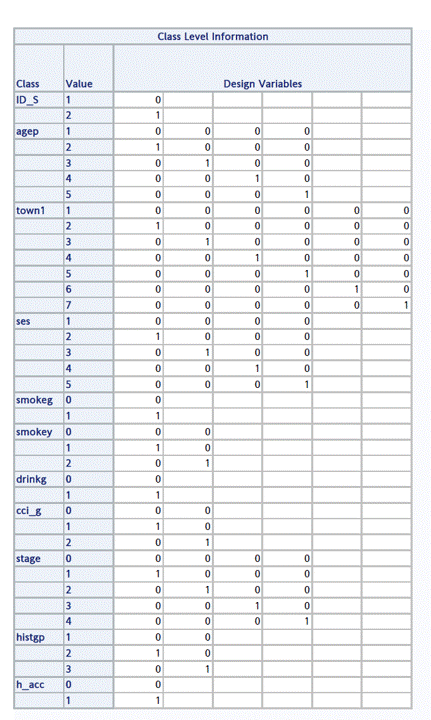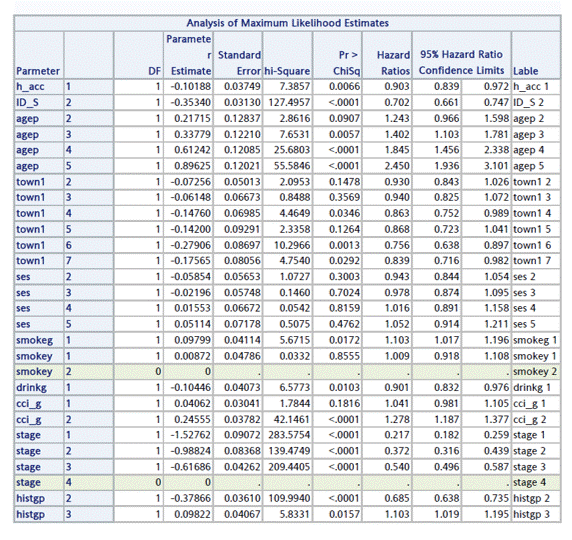## Cox PH model ：Hazard Ratios is the blank problem

Hello, I used the Cox PH model, but the output in the two rows with smokey=2 and stage=4 is blank, so I would like to ask how to solve the blank problem. (the logs do not show any warning)

Thank you !

``````proc phreg data=b;
class
ID_S (param=ref ref='1') agep(param=ref ref='1')
town1(param=ref ref='1')  ses (param=ref ref='1')
smokeg (param=ref ref='0') smokey (param=ref ref='0')
drinkg  (param=ref ref='0') cci_g (param=ref ref='0') stage (param=ref ref='0')
histgp (param=ref ref='1') h_acc (param=ref ref='0') ;
model dd*readmit1(0)=h_acc ID_S agep town1 ses smokeg smokey drinkg cci_g stage histgp/risklimits
slentry=0.25 slstay=0.15 details;
run;``````1 ACCEPTED SOLUTION

Accepted Solutions

2 REPLIES 2

## Re: Cox PH model ：Hazard Ratios is the blank problem

Thank you for your kind assistance.

Discussion stats
• 2 replies
• 276 views
• 0 likes
• 2 in conversation